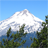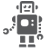# How to controll results?

cancel
Showing results for
Did you mean:
SOLVED

## How to controll results?

296 ViewsContributor II

Hi, I have to compute some values like current and resistance by software. I mean, I set a particular voltage from 0V to 3.3V ( so from 0 to 65534, for 16 bit) by software on a Digital to Analog Converter, then I want to compute the current, so I define the resistance (for example for 1 ohm resistance, I will write R= 19858, considering 16 bit, i Don't know if it is right) and then I compute the current I= voltage/R; Now, first of all, I don't know if it is possible to compute the current in this way, please, let me know how can I do. The second problem is that I want to verify this result, I mean, i want to veify if the current is right  and I want to see the value of I (the current), on arduino I used the serial port and I wrote printf ("current", I); and i could see the result, is  there a way to see the results on KDS? Thank you

Labels (1)
• ### General

1 Solution
197 ViewsNXP TechSupport

Hi,

i think your solution of calculating current is OK.

regarding how to see result with KDS, i suggest you use printf. here is an article for this topic,

Have a great day,
Zhang Jun

-----------------------------------------------------------------------------------------------------------------------
-----------------------------------------------------------------------------------------------------------------------

3 Replies
197 ViewsContributor II

Thank you Peter, I already did like you sugested and It works.

197 ViewsContributor II

Hello

Your calculation is a bit confusing (for me)

Here ist my way to solve that problem (if you can use float-values):

float R = 1;                                                             // [Ohm]

int16_t U_raw = acd_get_result();                    // replace with your function

float U = (float)U_raw  * ( 3.3 / 2^16 );           // [Volt] (or replace (3.3/2^16) with 0.00005)

float I = U / R;                                                       // [Ampere]

Peter

198 ViewsNXP TechSupport

Hi,

i think your solution of calculating current is OK.

regarding how to see result with KDS, i suggest you use printf. here is an article for this topic,